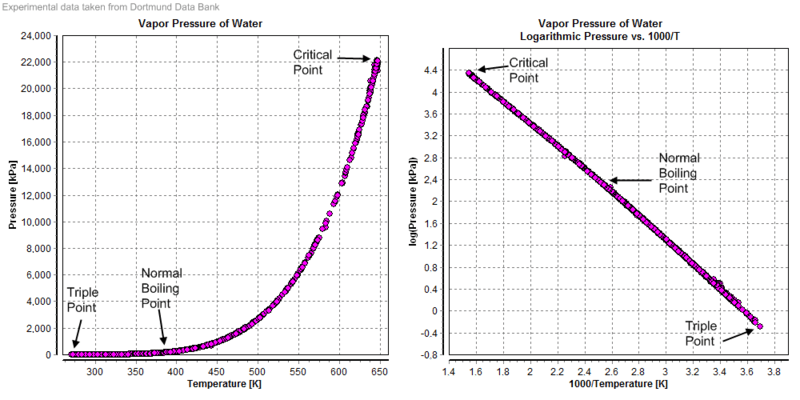# Difference Between Partial Pressure and Vapor Pressure

## Main Difference – Partial Pressure vs Vapor Pressure

Pressure is a force applied by a substance on a unit area of another substance. Partial pressure and vapor pressure are two types of pressure that are related to matter. The main difference between partial pressure and vapor pressure is that partial pressure is the pressure exerted by individual gases in a mixture of gases whereas vapor pressure is the pressure exerted by vapor which is in equilibrium with its condensed form.

### Key Areas Covered

1. What is Partial Pressure
– Definition, Characteristics, Calculation
2. What is Vapor Pressure
– Definition, Characteristics, Factors Affecting
3. What is the difference between Partial Pressure and Vapor Pressure
– Comparison of Key Differences

Key Terms: Pressure, Vapor, Mole Fraction, Partial Pressure, Vaporization, Volatile Compounds, Kinetic Energy## What is Partial Pressure?

The concept about the partial pressure was first proposed by the scientist John Dalton. It is defined as the pressure given by individual gases in a mixture of gases. Then the total pressure of that mixture of gases is the sum of partial pressures of every gas. Therefore, the partial pressure of a gas is always a lower value the total pressure of that system.

For example, the atmospheric pressure is the sum of the partial pressures of each and every gas found in the atmosphere. The partial pressure is calculated as below. If a gas named “X” is considered,

Partial pressure of X  =  mole fraction of X  *   Total pressure

Thus, the partial pressure of a gas is proportional to the mole fraction of the particular gas.Figure 01: Partial pressures of a mixture of Hydrogen (H2) and Helium (He) gases.

## What is Vapor Pressure?

Vapor pressure is the pressure exerted by vapor which is in equilibrium with its condensed form (liquid or solid phase). But when considering the vapor pressure, the system where the vapor exists should be a closed system with a constant temperature. Vapor pressure arises due to the escaping of liquid molecules (or solid) to the air of the closed system.

Since vapor pressure is related to the escaping of liquid molecules, substances with high vapor pressure at low temperatures are called volatile compounds. Formation of vapor is called vaporization. Vaporization can occur from both liquid and solid phases of matter.

Vapor pressure varies depending on the variations of temperature of a system. Increasing the temperature cause the rate of liquid molecules escaping the liquid surface to be increased. This occurs due to the increasing of kinesis (kinetic energy) of the liquid molecules. Hence, the vapor pressure also increases accordingly.Figure 02: Relationship between Vapor Pressure and Temperature

When it comes to the point where vapor pressure is equal to the external pressure inside that system, the liquid will boil.

### Factors Affecting the Vapor Pressure

#### Type of Molecules

The molecules which the solid or the liquid is composed of will affect the vapor pressure of a system. For example, if the interactions between molecules are stronger, the vapor pressure will be low because then it is difficult for the molecules to escape.

#### Temperature

If the temperature of the system is high, the vapor pressure will also be high, and if the temperature is low, then the vapor pressure will be low.

#### Surface Area

There is no effect of surface area on the vapor pressure.

## Difference Between Partial Pressure and Vapor Pressure

### Definition

Partial Pressure: Partial pressure is the pressure that arises from individual gases in a mixture of gases.

Vapor Pressure: Vapor pressure is the pressure exerted by vapor on its condensed form (either liquid or solid).

### Physical State

Partial Pressure: Partial pressure is related only to the gaseous phase.

Vapor Pressure: Vapor pressure is related to the solid and liquid phases.

### Volume

Partial Pressure: Partial pressure is calculated for gases which exist in the same volume.

Vapor Pressure: Vapor pressure does not depend on the volume of the system or the surface area of the solid or liquid.

### Mole Fraction

Partial Pressure: When calculating the partial pressure, the mole fraction of a particular gas is considered.

Vapor Pressure: In vapor pressure, the mole fraction of solute is considered because the solute molecules will form the vapor in that system.

### Conclusion

Partial pressure and vapor pressure are two terms which are used to determine the force exerted by a gaseous component towards the system which it is included. The main difference between partial pressure and vapor pressure is that partial pressure is the pressure of individual gases in a mixture of gases whereas vapor pressure is the pressure of vapor which is in equilibrium with its condensed form.

##### References:

1.”Vapor Pressure Formula.” Math. N.p., n.d. Web. Available here. 09 June 2017.
2. “Dalton’s Law of Partial Pressure – Boundless Open Textbook.” Boundless. Available here. 08 Aug. 2016. Web. 09 June 2017.

##### Image Courtesy:

1. “Presiones parciales” By Dr. Blair Jesse Ellyn Reich – (CC BY-SA 3.0) via Commons Wikimedia
2. “Vapor Pressure of Water” By WilfriedC – Own work (CC BY 3.0) via Commons Wikimedia